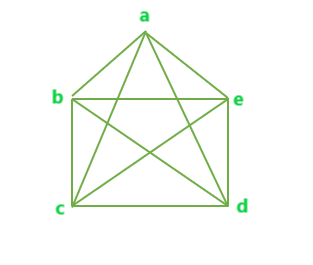Program to find total number of edges in a Complete Graph

• Difficulty Level : Basic
• Last Updated : 14 Apr, 2021

Given N number of vertices of a Graph. The task is to find the total number of edges possible in a complete graph of N vertices.
Complete Graph: A Complete Graph is a graph in which every pair of vertices is connected by an edge.
Examples

Input : N = 3
Output : Edges = 3

Input : N = 5
Output : Edges = 10

The total number of possible edges in a complete graph of N vertices can be given as,

Total number of edges in a complete graph of N vertices = ( n * ( n – 1 ) ) / 2

Example 1: Below is a complete graph with N = 5 vertices.The total number of edges in the above complete graph = 10 = (5)*(5-1)/2.
Below is the implementation of the above idea:

C++

 // C++ implementation to find the// number of edges in a complete graph #include using namespace std; // Function to find the total number of// edges in a complete graph with N verticesint totEdge(int n){    int result = 0;     result = (n * (n - 1)) / 2;     return result;} // Driver Codeint main(){    int n = 6;     cout << totEdge(n);     return 0;}

Java

 // Java implementation to find the// number of edges in a complete graph class GFG {     // Function to find the total number of// edges in a complete graph with N verticesstatic int totEdge(int n){    int result = 0;     result = (n * (n - 1)) / 2;     return result;}     // Driver Code    public static void main(String []args)    {        int n = 6;        System.out.println(totEdge(n));    } }

Python 3

 # Python 3 implementation to # find the number of edges# in a complete graph # Function to find the total# number of edges in a complete# graph with N verticesdef totEdge(n) :     result = (n * (n - 1)) // 2     return result             # Driver Codeif __name__ == "__main__" :     n = 6     print(totEdge(n)) # This code is contributed# by ANKITRAI1

C#

 // C# implementation to find// the number of edges in a// complete graphusing System; class GFG{     // Function to find the total// number of edges in a complete// graph with N verticesstatic int totEdge(int n){    int result = 0;     result = (n * (n - 1)) / 2;     return result;} // Driver Codepublic static void Main(){    int n = 6;    Console.Write(totEdge(n));}} // This code is contributed// by ChitraNayal



Javascript


Output:
15

My Personal Notes arrow_drop_up